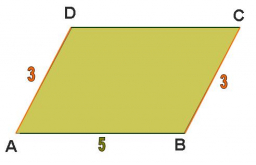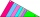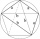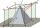# Parallelogram ABCD

We have the parallelogram ABCD, where AB is 6.2 cm
BC is 5.4 cm
AC is 4.8 cm
calculate the height on the AB side and the angle DAB

v =  4.0307 cm
A =  36.7385 °

### Step-by-step explanation:Did you find an error or inaccuracy? Feel free to write us. Thank you!Tips to related online calculators

#### You need to know the following knowledge to solve this word math problem:

We encourage you to watch this tutorial video on this math problem:

## Related math problems and questions:

• Squares above sidesTwo squares are constructed on two sides of the ABC triangle. The square area above the BC side is 25 cm2. The height vc to the side AB is 3 cm long. The heel P of height vc divides the AB side in a 2: 1 ratio. The AC side is longer than the BC side. Calc
• Parallelogram +ľ| AB | = 76cm, | BC | = 44cm, angle BAD = 30 °. Find the area of the parallelogram.
• ParallelogramFind the parallelogram's perimeter, where base a = 8 cm, height v = 3 cm, and angle alpha = 35° is the magnitude of the angle at vertex A.
• Triangle ABC v2Area of the triangle is 12 cm square. Angle ACB = 30º , AC = (x + 2) cm, BC = x cm. Calculate the value of x.
• Parallelogram ABCDThe area of parallelogram ABCD is 440 cm2. Points M and N are the midpoints of the sides AB and BC. What is the area of a quadrilateral MBND?
• ParallelogramCalculate area of the parallelogram ABCD as shown if |AB| = 19 cm, |BC| = 18 cm and angle BAD = 90°
• Triangle in a squareIn a square ABCD with side a = 6 cm, point E is the center of side AB, and point F is the center of side BC. Calculate the size of all angles of the triangle DEF and the lengths of its sides.
• TrapezoidsIn the isosceles trapezoid ABCD we know: AB||CD, |CD| = c = 8 cm, height h = 7 cm, |∠CAB| = 35°. Find the area of the trapezoid.
• Sin cos tanIn triangle ABC, right-angled at B. Sides/AB/=7cm, /BC/=5cm, /AC/=8.6cm. Find to two decimal places. A. Sine C B. Cosine C C. Tangent C.
• The basesThe bases of the isosceles trapezoid ABCD have lengths of 10 cm and 6 cm. Its arms form an angle α = 50˚ with a longer base. Calculate the circumference and content of the ABCD trapezoid.The quadrilateral ABCD is composed of two right triangles ABD and BCD. For side lengths: |AD| = 3cm, | BC | = 12cm, | BD | = 5cm. How many square centimeters (area) does the quadrilateral ABCD have? The angles DAB and DBC are right.In a right triangle ABC with a right angle at the apex C, we know the side length AB = 24 cm and the angle at the vertex B = 71°. Calculate the length of the legs of the triangle.The rectangular ABCD trapeze, whose AD arm is perpendicular to the AB and CD bases, has an area of 15 cm square. Bases have lengths AB = 6cm, CD = 4cm. Calculate the length of the AC diagonal.Find circumference and area of the rhumbline ABCD if the short side AD of which has a length of 5 cm, and the heel of the height from D leading to the AB side divides the AB side into two sections of 3 cm and 4 cm.The height of the regular quadrangular pyramid is 6 cm, the length of the base is 4 cm. What is the angle between the ABV and BCV planes?How many glass are needed to produce glass with base regular 5-gon if one base triangle in the base is 4.2 square cm and the height is 10 cm?In a regular quadrilateral pyramid, the height is 6.5 cm and the angle between the base and the side wall is 42°. Calculate the surface area and volume of the body. Round calculations to 1 decimal place.